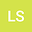•We study the existence and asymptotic behavior of least energy sign-changing solutions for the nonlinear Schr\“{o}dinger equation coupled with the Chern-Simons gauge theory $\left\{ \begin{gathered} -\Delta u+ \omega u+\lambda \sum_{j=1}^k\bigg( \frac{h^2(|x|)}{|x|^2}u^{2(j-1)} +\frac{1}{j}\int_{|x|}^\infty \frac{h(s)}{s}u^{2j}(s) ds \bigg)u= f(u) \ \ \text{in}\ \ \mathbb{R}^2 , \hfill \\ {\text{ }}u \in {H^1_r}({\mathbb{R}^2}), \hfill \\ \end{gathered} \right.$ where $\omega, ~\lambda >0$ are constants, $k\in \mathbb{N}^+$ and $h(s)=\int_0^s\frac{r}{2}u^2(r)dr.$ Under some suitable assumptions on $f\in C(\R)$, with the help of the Gagliardo-Nirenberg inequality, we apply the constraint minimization argument to obtain a least energy sign-changing solution $u_\lambda$ with precisely two nodal domains. Furthermore, we prove that the energy of $u_\lambda$ is strictly larger than two times of the ground state energy and analyze the asymptotic behavior of $u_\lambda$ as $\lambda\searrow0^+$. Our results cover and improve the existing ones for the gauged nonlinear Schr\”{o}dinger equation when $k\equiv1$.